# Machine Learning#

## Matrices#

mlstatpy.ml.matrices.gram_schmidt (mat, change = False)

Applies the Gram–Schmidt process. Due to performance, every row is considered as a vector.

mlstatpy.ml.matrices.linear_regression (X, y, algo = None)

Solves the linear regression problem, find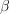which minimizes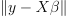, based on the algorithm Arbre de décision optimisé pour les régressions linéaires.

Updates matrix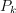to produce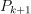which is the matrix P in algorithm Streaming Linear Regression. The function modifies the matrix Pk given as an input.

mlstatpy.ml.matrices.streaming_gram_schmidt (mat, start = None)

Solves the linear regression problem, findwhich minimizes, based on algorithm Streaming Gram-Schmidt.

Updates coefficients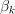to produce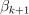in Streaming Linear Regression. The function modifies the matrix Pk given as an input.

mlstatpy.ml.matrices.streaming_linear_regression (mat, y, start = None)

Streaming algorithm to solve a linear regression. See Streaming Linear Regression.

Updates coefficientsto producein Streaming Linear Regression. The function modifies the matrix Pk given as an input.

mlstatpy.ml.matrices.streaming_linear_regression_gram_schmidt (mat, y, start = None)

Streaming algorithm to solve a linear regression with Gram-Schmidt algorithm. See Streaming Linear Regression version Gram-Schmidt.

## Métriques#

mlstatpy.ml.MlGridBenchMark (self, name, datasets, clog = None, fLOG = <function noLOG at 0x7fb8b202bb80>, path_to_images = “.”, cache_file = None, progressbar = None, graphx = None, graphy = None, params)

The class tests a list of model over a list of datasets.

mlstatpy.ml.ROC (self, y_true = None, y_score = None, sample_weight = None, df = None)

Helper to draw a ROC curve.

mlstatpy.ml.voronoi_estimation_from_lr (L, B, C = None, D = None, cl = 0, qr = True, max_iter = None, verbose = False)

Determines a Voronoi diagram close to a convex partition defined by a logistic regression in n classes.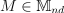a row matrix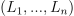. Every border between two classes i and j is defined by: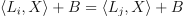.

The function looks for a set of points from which the Voronoi diagram can be inferred. It is done through a linear regression with norm L1. See Régression logistique, diagramme de Voronoï, k-Means.

## Plus proches voisins#

Définit une classe de nuage de points. On suppose qu’ils sont définis par une matrice, chaque ligne est un élément.

mlstatpy.ml.kppv_laesa.NuagePointsLaesa (self, nb_pivots)

Implémente l’algorithme des plus proches voisins, version :ref:LAESA <space_metric_algo_laesa_prime>_

## Tree and neural networks#

mlstatpy.ml._neural_tree_node.NeuralTreeNode (self, weights, bias = None, activation = “sigmoid”, nodeid = -1, tag = None)

One node in a neural network.

mlstatpy.ml.neural_tree.NeuralTreeNet (self, dim, empty = True)

Node ensemble.

mlstatpy.ml.neural_tree.BaseNeuralTreeNet (self, estimator, optimizer = None, max_iter = 100, early_th = None, verbose = False, lr = None, lr_schedule = None, l1 = 0.0, l2 = 0.0, momentum = 0.9)

Classifier or regressor following scikit-learn API.

mlstatpy.ml.neural_tree.NeuralTreeNetClassifier (self, estimator, optimizer = None, max_iter = 100, early_th = None, verbose = False, lr = None, lr_schedule = None, l1 = 0.0, l2 = 0.0, momentum = 0.9)

Classifier following scikit-learn API.

mlstatpy.ml.neural_tree.NeuralTreeNetRegressor (self, estimator, optimizer = None, max_iter = 100, early_th = None, verbose = False, lr = None, lr_schedule = None, l1 = 0.0, l2 = 0.0, momentum = 0.9)

Regressor following scikit-learn API.